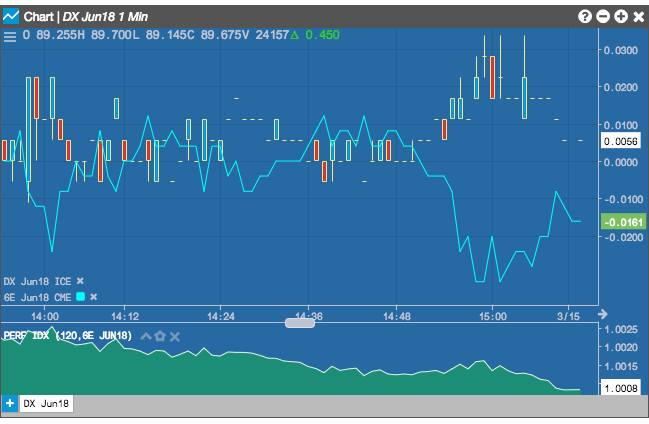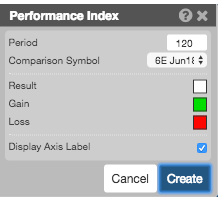Charts

# Performance Index

The Performance Index indicator is used to compare the price trend of one instrument with the performnance of a benchmark index.

Note: The Performance Index is valid only when comparing multiple instruments using either Comparisons or Series. When more that two instruments are used, each correlation indicator is compared to the chart's first instrument.## Configuration Options• Period: Number of bars to use in the calculations.
• Comparison Symbol: Instrument from the chart to compare.
• Color Selectors: Colors to use for graph elements.

## Formula

$PI = \frac {Instr\ Close}{Index\ Close} \times \frac {Index \ MA}{Instr \ MA}$

where MA is the moving average.